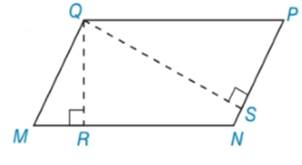Chapter 5.3, Problem 37EElementary Geometry For College St...

7th Edition
Alexander + 2 others
ISBN: 9781337614085

Solutions

Chapter
SectionElementary Geometry For College St...

7th Edition
Alexander + 2 others
ISBN: 9781337614085
Textbook Problem

Use the result of Exercise 13 to do the following problem. In ▱ M N P Q , Q P = 12 and Q M = 9 . The length of altitude Q R ¯ (to side M N ¯ ) is 6. Find the length of altitude Q S ¯ from Q to P N ¯ .To determine

To find:

The length of altitude QS¯ from Q to PN¯.

Explanation

Given:

Given: MNPQ, QP=12, QM=9, and the length of altitude QR¯ (to side MN¯) is 6.

Definition:

AA:

If the two angles of one triangle are congruent to the two angles of another triangle, then the triangles are similar.

CSSTP:

Corresponding sides of similar triangles are proportional.

Description:

Given that MNPQ,.

From the given figure, it is observed that there are two altitudes QR¯ and QS¯.

An altitude is a line segment through a vertex and perpendicular to a line of a base.

That is, QR¯MN¯ and QS¯PN¯.

It is known that two lines are perpendicular, they form right angles. That is, QRM and QSP are right triangles.

Since all right angles are congruent, QRMQSP.

The opposite angles of are congruent. So that RS.

The above mentioned AA definition, the two triangles QRM and QSP are similar since the two angles of one triangle are congruent to two angles of another triangle

Still sussing out bartleby?

Check out a sample textbook solution.

See a sample solution

The Solution to Your Study Problems

Bartleby provides explanations to thousands of textbook problems written by our experts, many with advanced degrees!

Get Started

Evaluate the improper integrals in Problems 31-34. 33.

Mathematical Applications for the Management, Life, and Social Sciences

Explain what happens during each of the two stages of the two-factor ANOVA.

Essentials of Statistics for The Behavioral Sciences (MindTap Course List)

find the real roots of each equation by factoring. 133. 14x2x+1=0

Applied Calculus for the Managerial, Life, and Social Sciences: A Brief Approach

Evaluate the integral 30. dxex1e2x

Single Variable Calculus: Early Transcendentals

The gradient vector field for is:

Study Guide for Stewart's Multivariable Calculus, 8th